# Catch up on the latest AI articles# Fine-tuning A Text-to-image Model Using Human Evaluation Feedback

3 main points
✔️ Propose a method for fine-tuning a text-to-image model using feedback from human evaluations
✔️ The reward function is trained by asking people to evaluate examples generated in response to prompts. The obtained reward function is used to update the image generation model.
✔️ The proposed method can generate images that more accurately reflect the number of objects, colors, backgrounds, etc. in the prompt.

Aligning Text-to-Image Models using Human Feedback
written by Kimin LeeHao LiuMoonkyung RyuOlivia WatkinsYuqing DuCraig BoutilierPieter AbbeelMohammad GhavamzadehShixiang Shane Gu
(Submitted on 23 Feb 2023)

Subjects:  Machine Learning (cs.LG); Artificial Intelligence (cs.AI); Computer Vision and Pattern Recognition (cs.CV)code：The images used in this article are from the paper, the introductory slides, or were created based on them.

## Introduction

Although the method of generating images based on textual instructions (prompts) has been greatly developed in recent years, it is sometimes problematic to generate images that are not well aligned with the instructions.

In the context of language models, RLHF is emerging as a method of learning based on human feedback and aligning model behavior with human values.

The method first uses human evaluations of the model's output to learn a reward function, which is then used to optimize the language model through reinforcement learning.

The paper presented here attempts to perform fine-tuning of text-to-image models using the reward function. (Note that the method presented here is not strictly RLHF, since it does not use reinforcement learning.)

An overview of the proposed method is shown in the following figure.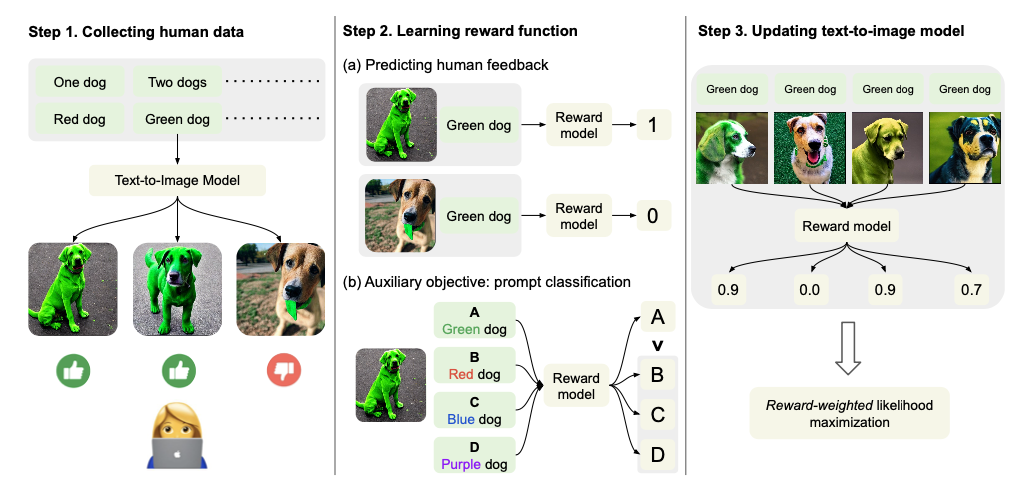(1) First, have the system generate a variety of images in response to text prompts and collect feedback on the human evaluation.

(2) Next, we train the reward function to predict human ratings using the obtained data. In addition to the usual task of predicting ratings, we train the reward function for an alternative task: identifying the prompts used to generate the images.

(3) Then, while using the reward function, the model is updated in the semi-supervised learning fashion, unlike the usual method using reinforcement learning (RL).

In this study, the stable diffusion model [Rombach et al., 2022] is used as the image generation model.

## Technique

Steps (1)~(3) described above are explained in detail.

### (1) Collection of human evaluation data

Generate up to 60 images per prompt using the Stable diffusion model. The prompts specify the number of images, color, and background. Example: two green dogs in a city

Because of the simplicity of the prompt request, human ratings are obtained with binary labels: good or bad.

### (2) Learning reward functions

We train a function $r_{\phi}$ that takes an image $x$ and a prompt $z$ as input and outputs the predicted value $y$ of the human evaluation. The predictions are 1 for "good" and 0 for "bad". The objective function of the reward function learning is as follows.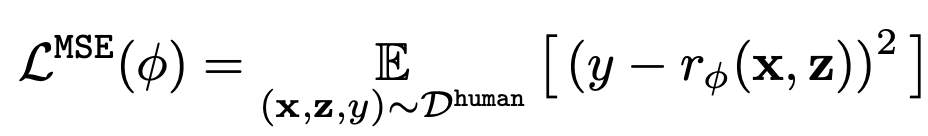Another objective function is added in terms of data expansion.

A dummy prompt is prepared in which one part of the prompt $Z$ is replaced by another, and the task is to correctly select the original prompt, using the following objective function.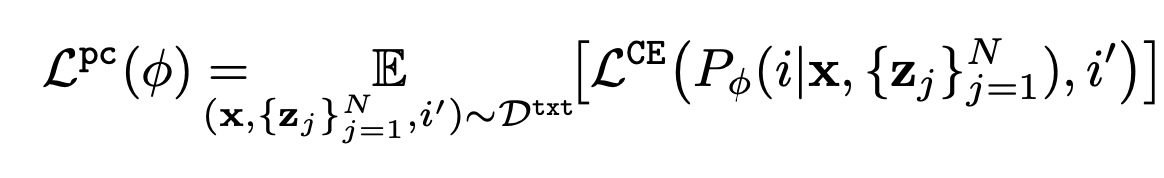However, $P_{\phi}$ represents the selection probability of the prompt.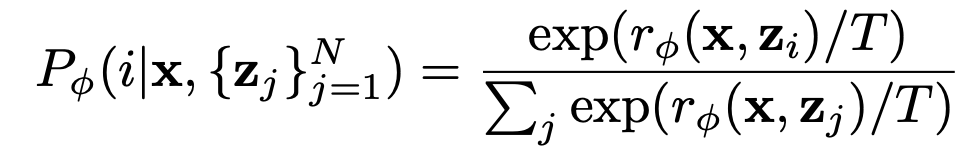The final objective function is the combination of the above two objective functions.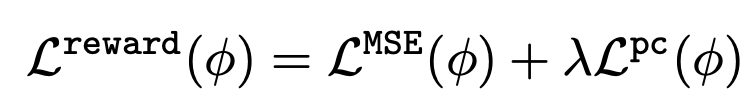### (3) Fine-tuning of text-to-image model

Using the learned reward function, the text-to-image model is fine-tuned by the following equation based on minimizing the negative log-likelihood.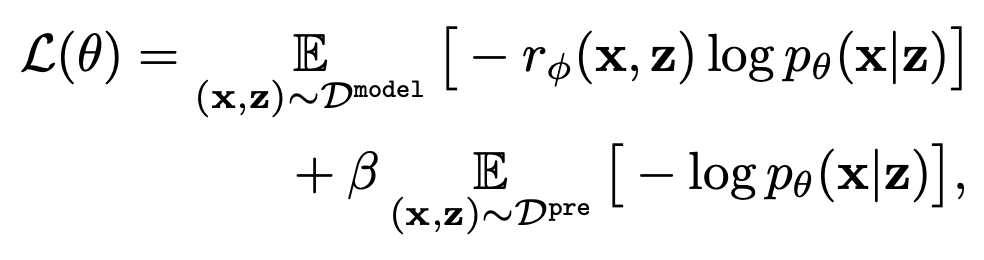The first term has the effect of bringing the generated results of the model closer to following the prompts. The second term ensures the diversity of the generated results.

## Experiment

### Model Setting

Based on the stable diffusion model, the CLIP part is frozen during fine-tuning, and only the diffusion module part is trained.

The reward function model is the ViT-L/14 CLIP model [Radford et al., 2021], which computes image and text embeddings and returns a score by MLP.

### Evaluation by People

Two images are presented to the raters, one generated by the proposed model (fine-tuning model) and the other by the original stable diffusion model, and the raters are asked which of the two models is more likely to follow the prompt instructions. Nine raters' ratings are collected for each pair. The results are shown on the left in the next figure.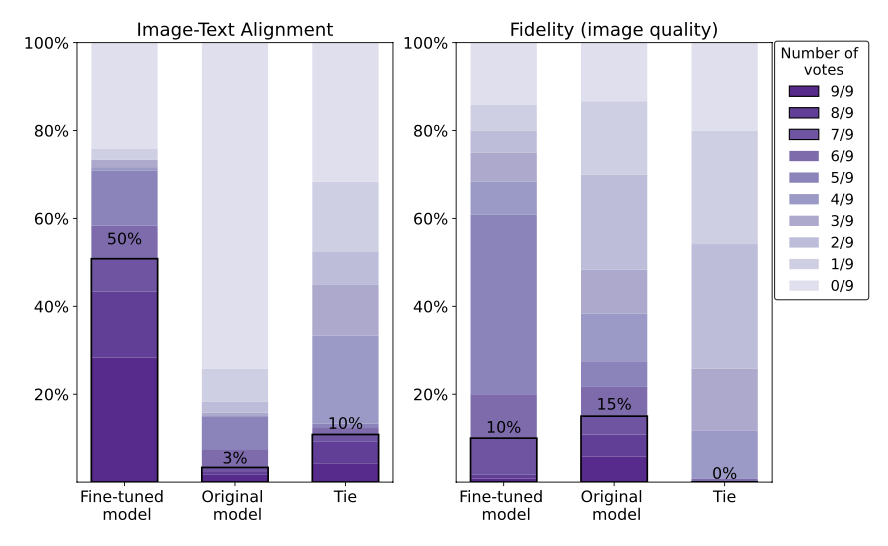The results show that the images produced by the proposed model (fine-tuned model) have a better fit to the text than the original model. On the other hand, the quality of the images (right figure) shows a slight decrease in performance. This may be due to the amount of data used for fine-tuning or the fact that only text-fit was used for evaluation.

### Qualitative evaluation

Let's qualitatively compare the image generation of the proposed model with that of the stable diffusion model. The results are as follows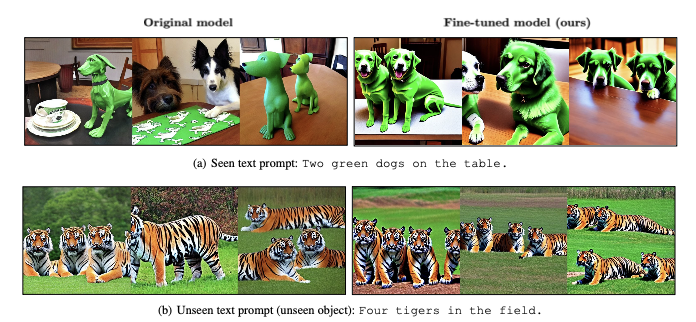This shows that the proposed model is able to accurately reflect instructions by number of pieces, color, and background.

On the other hand, however, some problems were observed, such as a decrease in image diversity. The authors state that this problem can be overcome by increasing the amount of data.

## Discussion

In this paper, we propose a method to improve the behavior of the image-to-text model through fine-tuning using feedback from human evaluations, and to generate images that accurately follow prompt instructions on the number of pieces, color, background, and so on. Experiments revealed that there is a trade-off between following prompt instructions and ensuring image quality (e.g., diversity), which is difficult to reconcile.

At the end of the paper, he mentioned the possibility of future development. For example, in this experiment, the accuracy was evaluated by humans within a limited range, such as number of pieces and color.

This paper is the first step in an effort to improve text-to-image models using human evaluation feedback. We look forward to further development of this research in the future.

If you have any suggestions for improvement of the content of the article,